## 6-2 EPD in Time Domain (嚙踝蕭嚙踝蕭嚙踝蕭嚙踝蕭嚙踝蕭嚙踝蕭嚙踝蕭嚙踝蕭嚙踝蕭嚙踝蕭嚙踝蕭嚙踝蕭嚙踝蕭嚙踝蕭嚙踝蕭)

[chinese][all]

We shall introduce several time-domain methods for EPD in this section.

The first method uses volume as the only acoustic feature for EPD. This is the most intuitive method with least computation. We only need to determine a volume threshold. Any frame with a volume less than the threshold is regarded as silence. However, how to determine a good volume threshold is not obvious. As a result, the best strategy is to use a set of labeled training data to find the best value for achieving the minimum error.

Hint
When computing volume, remember to perform zero justification first.

In the following example, we shall use four different ways to compute volume thresholds for EPD of the wave file sunday.wav:

Example 1: epdByVolTh01.mwaveFile='sunday.wav'; au=myAudioRead(waveFile); y=au.signal; fs=au.fs; frameSize = 256; overlap = 128; y=y-mean(y); % zero-mean substraction frameMat=buffer2(y, frameSize, overlap); % frame blocking frameNum=size(frameMat, 2); % no. of frames volume=frame2volume(frameMat); % volume volumeTh1=max(volume)*0.1; % volume threshold 1 volumeTh2=median(volume)*0.1; % volume threshold 2 volumeTh3=min(volume)*10; % volume threshold 3 volumeTh4=volume(1)*5; % volume threshold 4 index1 = find(volume>volumeTh1); index2 = find(volume>volumeTh2); index3 = find(volume>volumeTh3); index4 = find(volume>volumeTh4); endPoint1=frame2sampleIndex([index1(1), index1(end)], frameSize, overlap); endPoint2=frame2sampleIndex([index2(1), index2(end)], frameSize, overlap); endPoint3=frame2sampleIndex([index3(1), index3(end)], frameSize, overlap); endPoint4=frame2sampleIndex([index4(1), index4(end)], frameSize, overlap); subplot(2,1,1); time=(1:length(y))/fs; plot(time, y); ylabel('Amplitude'); title('Waveform'); axis([-inf inf -1 1]); line(time(endPoint1( 1))*[1 1], [-1, 1], 'color', 'm'); line(time(endPoint2( 1))*[1 1], [-1, 1], 'color', 'g'); line(time(endPoint3( 1))*[1 1], [-1, 1], 'color', 'k'); line(time(endPoint4( 1))*[1 1], [-1, 1], 'color', 'r'); line(time(endPoint1(end))*[1 1], [-1, 1], 'color', 'm'); line(time(endPoint2(end))*[1 1], [-1, 1], 'color', 'g'); line(time(endPoint3(end))*[1 1], [-1, 1], 'color', 'k'); line(time(endPoint4(end))*[1 1], [-1, 1], 'color', 'r'); legend('Waveform', 'Boundaries by threshold 1', 'Boundaries by threshold 2', 'Boundaries by threshold 3', 'Boundaries by threshold 4'); subplot(2,1,2); frameTime=frame2sampleIndex(1:frameNum, frameSize, overlap); plot(frameTime, volume, '.-'); ylabel('Sum of Abs.'); title('Volume'); axis tight; line([min(frameTime), max(frameTime)], volumeTh1*[1 1], 'color', 'm'); line([min(frameTime), max(frameTime)], volumeTh2*[1 1], 'color', 'g'); line([min(frameTime), max(frameTime)], volumeTh3*[1 1], 'color', 'k'); line([min(frameTime), max(frameTime)], volumeTh4*[1 1], 'color', 'r'); legend('Volume', 'Threshold 1', 'Threshold 2', 'Threshold 3', 'Threshold 4');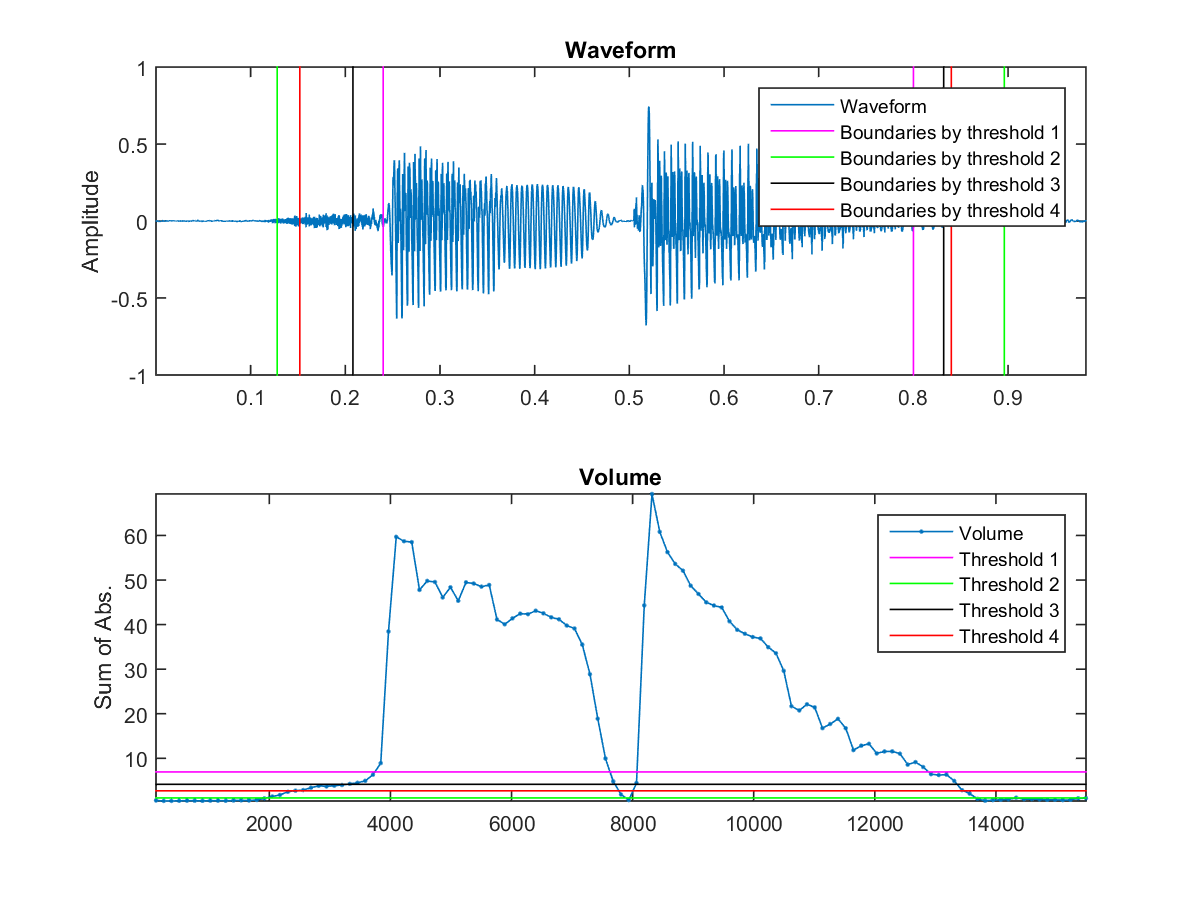In the above example, we have used four methods to compute the volume thresholds. These four methods all have their weakness, as explained next:

1. A ratio times the maximal volume: Not reliable if there is an impulse in volume due to plosive sounds.
2. A ratio times the median of the volume: Not reliable when silence occupies more than half of the audio signals.
3. The minimal volume times a constant: This could go wrong if the noise if too big. Moreover, it is likely for some recordings to have a frame of zero volume.
4. The volume of the first frame times a constant: This could go wrong if the first frame of the recording is unstable, which is not rare in practice.
Since there is no simply way to choose a volume threshold for EPD of all kinds of recordings, all we can expect is look into more sophisticated methods for determining the threshold. A more robust example of EPD is shown next.

Example 2: epdByVol01.mwaveFile='singaporeIsAFinePlace.wav'; au=myAudioRead(waveFile); opt=endPointDetect('defaultOpt'); opt.method='vol'; showPlot=1; endPoint=endPointDetect(au, opt, showPlot);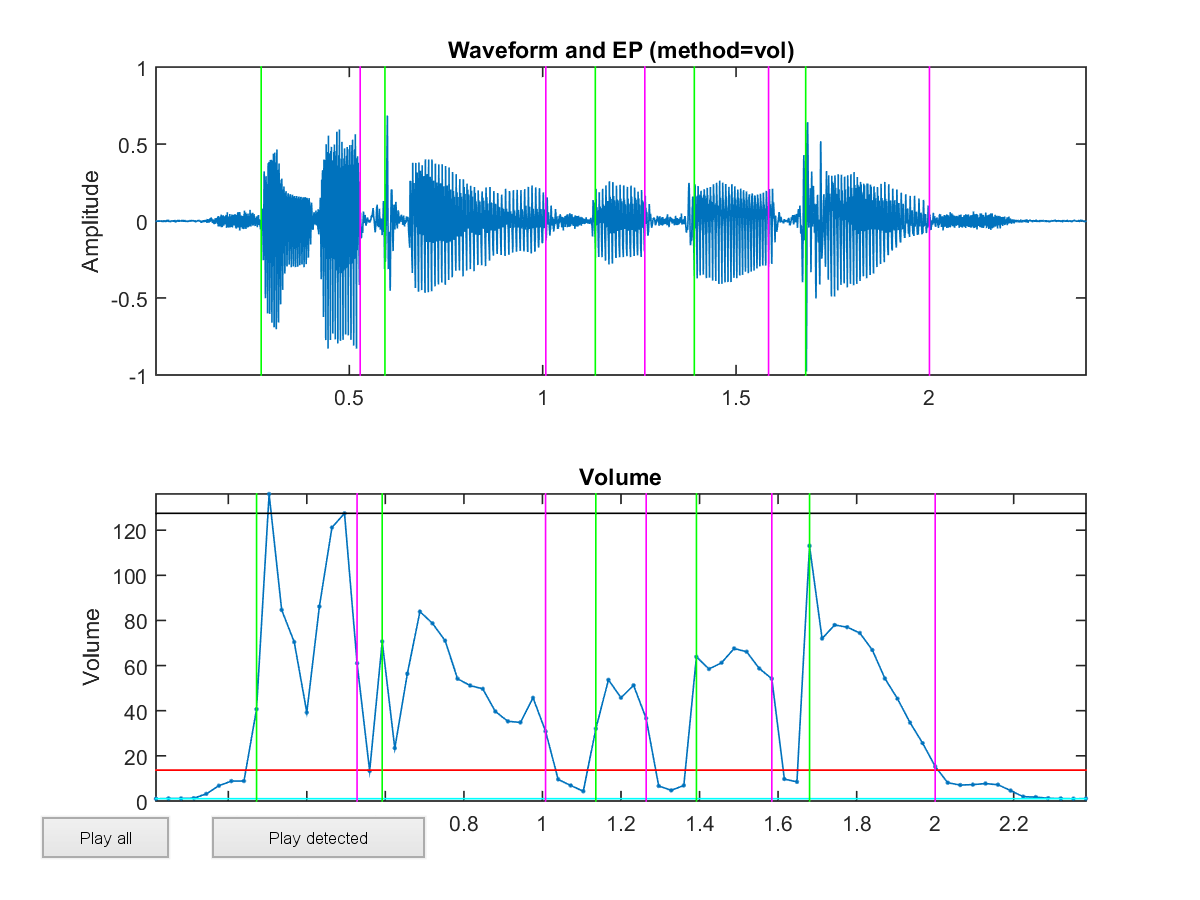In the above example, the volume threshold is determined as
volTh=(volMax-volMin)*epdPrm.volRatio+volMin;
where epdPrm.volRatio is 0.1, and volMin and volMax are 3% and 97% percentiles of the volumes in an utterance, respectively.

The ratios or constants in the above four methods should be determined through labeled training data. It should be noted that wave files of different characteristics (recordings via uni-directional or omni-directional microphones, different sample rates, different bit resolutions, different frame sizes and overlaps) will have a different best thresholds.

Of course, you also create a new threshold by using linear combinations of these thresholds, etc.

From the above example, it is obvious that the leading unvoiced sound is likely to be misclassified as silence. Moreover, a single threshold might not perform well if the volume varies a lot. As a result, an improved method can be stated next:

1. Use a upper threshold tu to determine the inital end-points.
2. Extend the boundaries until they reach the lower threshold tl.
3. Extend the boundaries further until they reach the ZCR threshold tzc.
This method is illustrated as follows.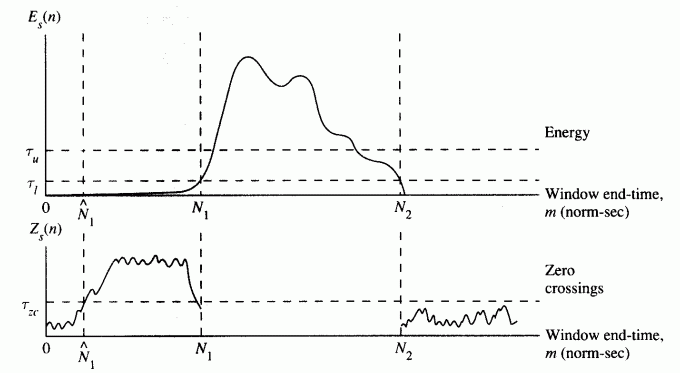The above improved method uses only three thresholds, hence it is possible to use grid search to find the best values via a set of labeled training data.

Hint
The above method is designed for speech recognition. For melody recognition, we do not need to consider unvoiced sounds since they do not have pitch at all.

If we apply the above method for EPD of sunday.wav, the result can plotted as follows:

Example 3: epdByVolZcr01.mwaveFile='singaporeIsAFinePlace.wav'; au=myAudioRead(waveFile); opt=endPointDetect('defaultOpt'); opt.method='volZcr'; showPlot=1; endPoint=endPointDetect(au, opt, showPlot);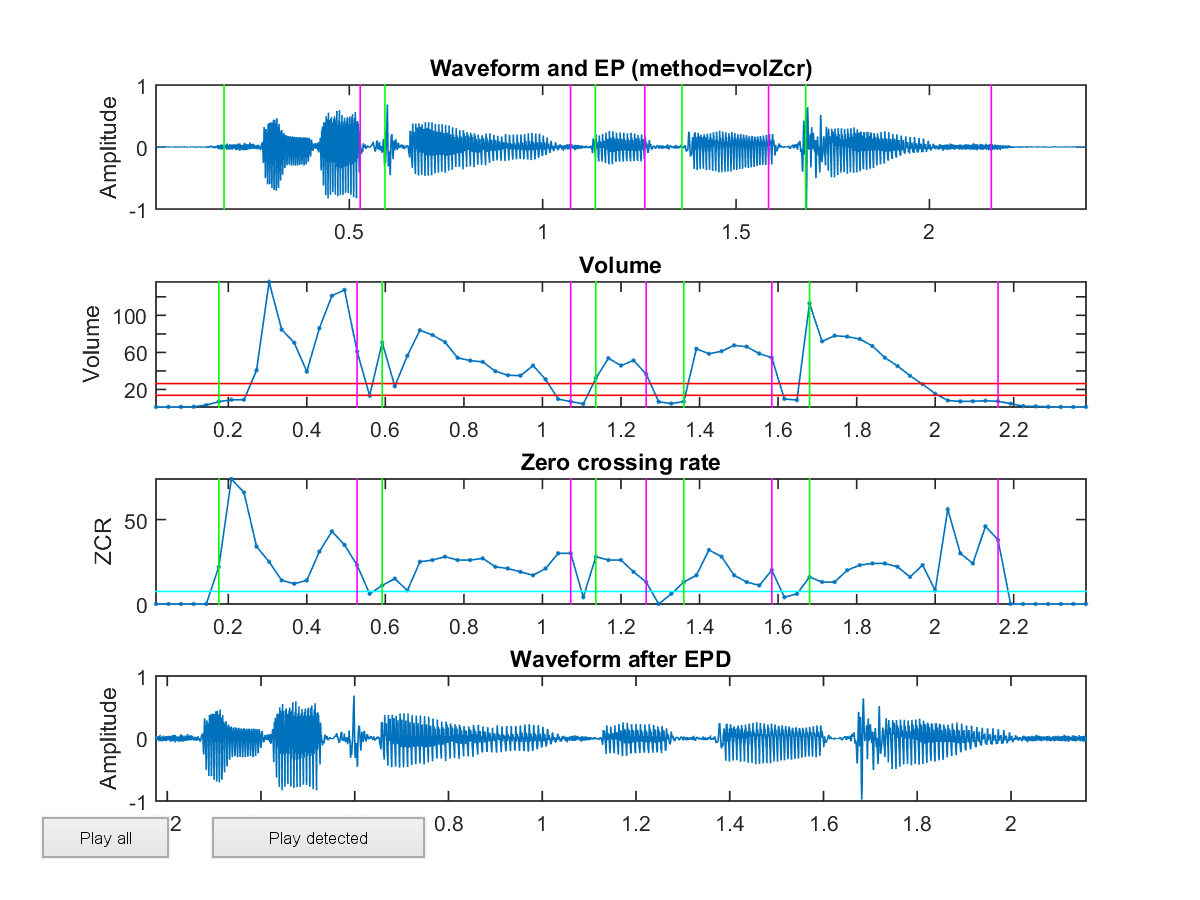In the above plot, the red and green lines indicate the beginning and end of sound, respectively. This example uses the function epdByVolZcr.m in the SAP Toolbox, which use volume and ZCR as mentioned above for EPD.

Now it should be obvious that the most difficult part in EPD is to distinguish unvoiced sounds from silence reliably. One way to achieve this goal is to use high-order difference of the waveform as a time-domain features. For instance, in the following example, we use order-1, 2, 3 differences on the waveform of beautifulSundays.wav:

It is obvious that the high-order difference (HOD) on the waveform can let us identify the unvoiced sound more easily for this case. Therefore you can take the union of high-volume and high HOD regions to have most robust of EPD.

Example 4: highOrderDiff01.mwaveFile='singaporeIsAFinePlace.wav'; au=myAudioRead(waveFile); y=au.signal; fs=au.fs; frameSize = 256; overlap = 128; y=y-mean(y); % zero-mean substraction frameMat=buffer2(y, frameSize, overlap); % frame blocking frameNum=size(frameMat, 2); % no. of frames volume=frame2volume(frameMat); sumAbsDiff1=sum(abs(diff(frameMat))); sumAbsDiff2=sum(abs(diff(diff(frameMat)))); sumAbsDiff3=sum(abs(diff(diff(diff(frameMat))))); sumAbsDiff4=sum(abs(diff(diff(diff(diff(frameMat)))))); subplot(2,1,1); time=(1:length(y))/fs; plot(time, y); ylabel('Amplitude'); title('Waveform'); subplot(2,1,2); frameTime=frame2sampleIndex(1:frameNum, frameSize, overlap)/fs; plot(frameTime', [volume; sumAbsDiff1; sumAbsDiff2; sumAbsDiff3; sumAbsDiff4]', '.-'); legend('Volume', 'Order-1 diff', 'Order-2 diff', 'Order-3 diff', 'Order-4 diff'); xlabel('Time (sec)');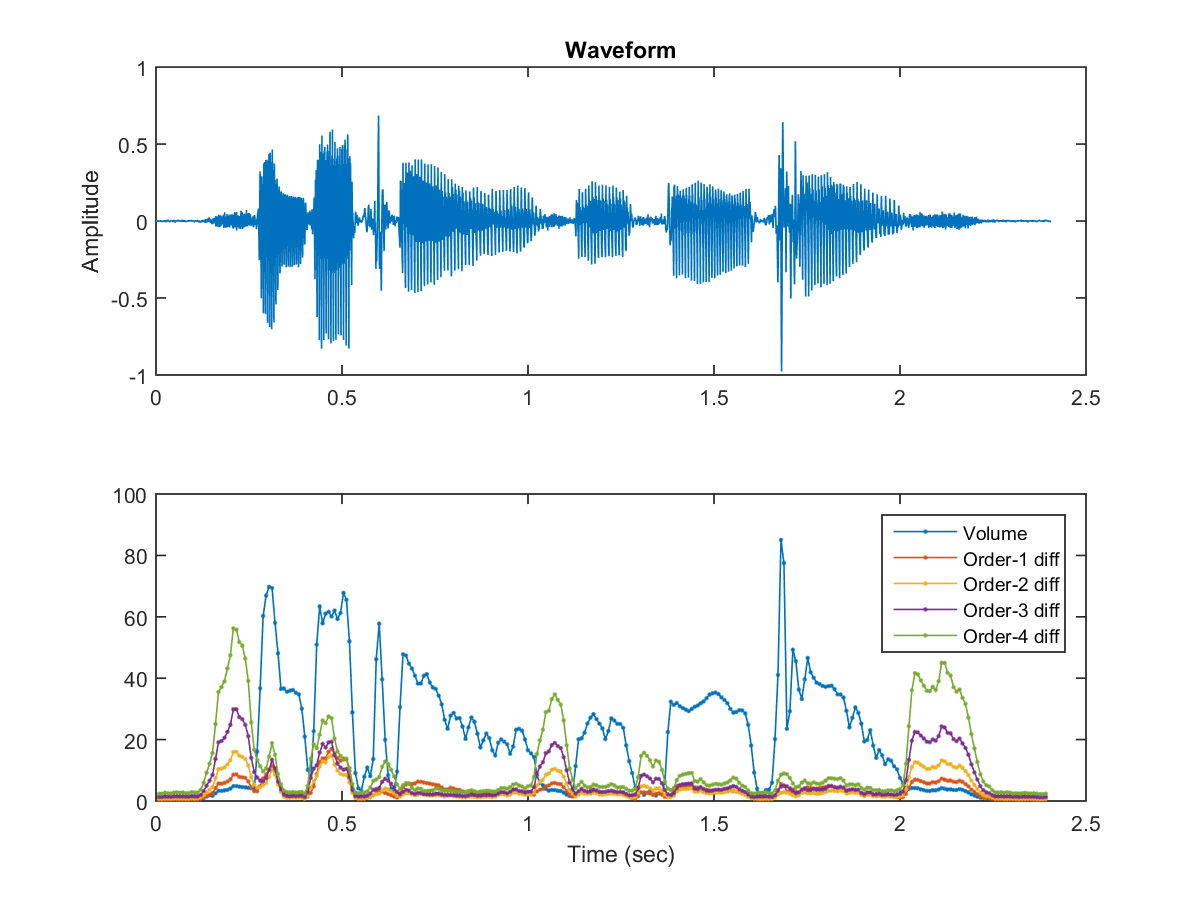From the above example, a possible simple way of combining volume and HOD for EPD can be stated as follows:

1. Compute volume (VOL) and the absolute sum of order-n difference (HOD).
2. Select a weighting factor w within [0, 1] to compute a new curve VH = w*VOL + (1-w)*HOD.
3. Find a ratio r to compute the threshold t of VH to determine the end-points. The threshold is equal to VHmin+(VHmax-VHmin)*r.

The above method involves three parameters to be determined: n, w, r. Typical values of these parameters are n = 1, w = 0.76, and r = 0.012. However, these values vary with data sets. It is always advisable to have these values tuned by using the target data set for a more robust result.

A more comprehensive version of EPD using volume and HOD is epdByVolHod.m in the SAP toolbox. Please see the following example.

Example 5: epdByVolHod01.mwaveFile='singaporeIsAFinePlace.wav'; au=myAudioRead(waveFile); opt=endPointDetect('defaultOpt'); opt.method='volHod'; showPlot=1; endPoint=endPointDetect(au, opt, showPlot);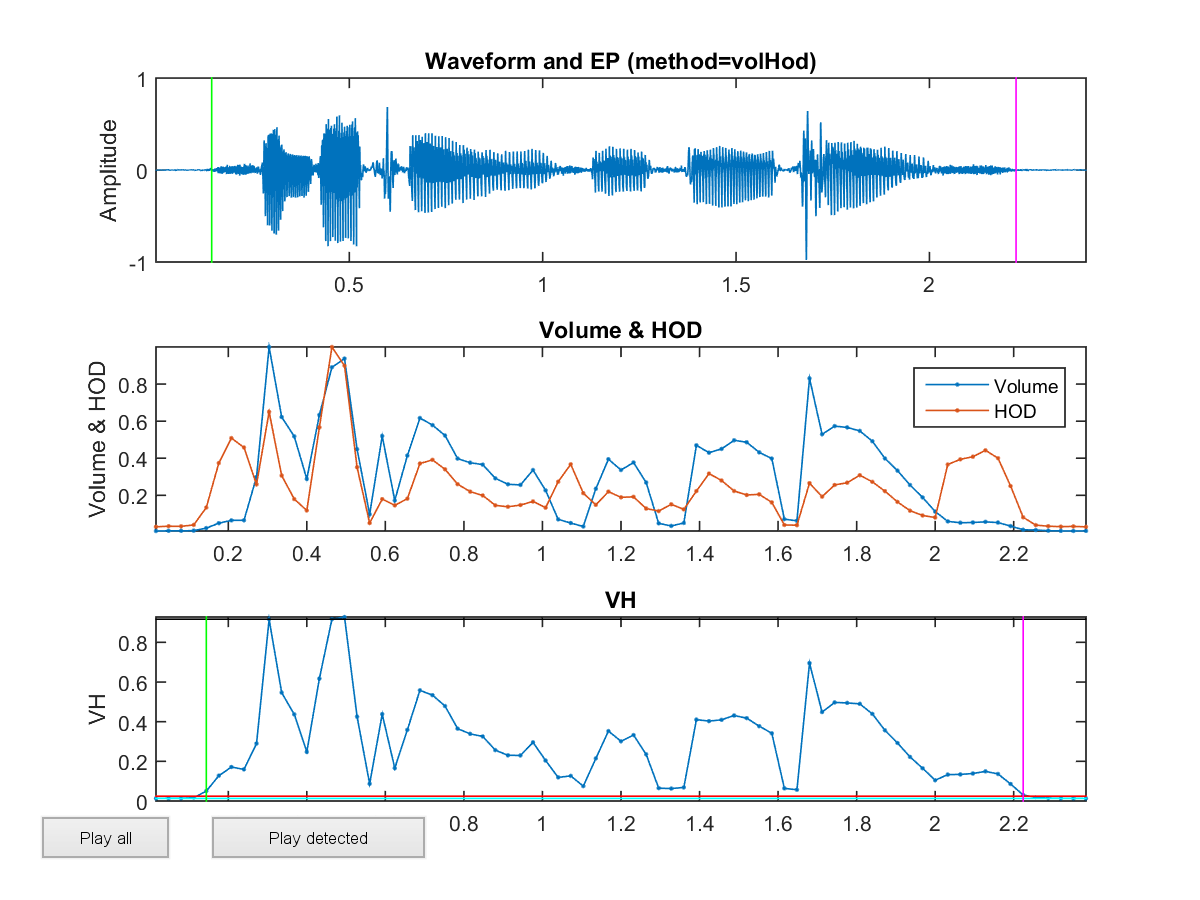In general, the joint use of volume and HOD can perform decent EPD for most recordings, except for those with high environmental noise.

Of course, there are still plenty of other methods for EPD on time domain. It is up to you to create ingenious methods for EPD.

Audio Signal Processing and Recognition (音訊處理與辨識)